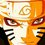# Olympiad Proof Problem - Day 7

1. Find all prime numbers $p_{1}$, $p_{2}$, $p_{3}$ $\ldots$ $p_{n}$ such that $\prod_{i=1}^{n} p_{i} = 10 \sum_{i=1}^{n} p_{i}$

2. Given a quadrilateral $ABCD$ with $\angle B=\angle D=90^{\circ}$. Point $M$ is chosen on segment $AB$ so that $AD=AM$. Rays $DM$ and $CB$ intersect at point $N$. Points $H$ and $K$ are feet of perpendiculars from points $D$ and $C$ to lines $AC$ and $AN$ respectively. Prove that $\angle MHN=\angle MCK$.

###### Try more proof problems at Olympiad Proof Problems.Note by Surya Prakash
4 years, 2 months ago

This discussion board is a place to discuss our Daily Challenges and the math and science related to those challenges. Explanations are more than just a solution — they should explain the steps and thinking strategies that you used to obtain the solution. Comments should further the discussion of math and science.

When posting on Brilliant:

• Use the emojis to react to an explanation, whether you're congratulating a job well done , or just really confused .
• Ask specific questions about the challenge or the steps in somebody's explanation. Well-posed questions can add a lot to the discussion, but posting "I don't understand!" doesn't help anyone.
• Try to contribute something new to the discussion, whether it is an extension, generalization or other idea related to the challenge.

MarkdownAppears as
*italics* or _italics_ italics
**bold** or __bold__ bold
- bulleted- list
• bulleted
• list
1. numbered2. list
1. numbered
2. list
Note: you must add a full line of space before and after lists for them to show up correctly
paragraph 1paragraph 2

paragraph 1

paragraph 2

[example link](https://brilliant.org)example link
> This is a quote
This is a quote
    # I indented these lines
# 4 spaces, and now they show
# up as a code block.

print "hello world"
# I indented these lines
# 4 spaces, and now they show
# up as a code block.

print "hello world"
MathAppears as
Remember to wrap math in $$ ... $$ or $ ... $ to ensure proper formatting.
2 \times 3 $2 \times 3$
2^{34} $2^{34}$
a_{i-1} $a_{i-1}$
\frac{2}{3} $\frac{2}{3}$
\sqrt{2} $\sqrt{2}$
\sum_{i=1}^3 $\sum_{i=1}^3$
\sin \theta $\sin \theta$
\boxed{123} $\boxed{123}$

Sort by:

Q1) A bit of a sleepy proof (I'm gonna sleep soon). Tell me my errors and I'll rectify them.

Firstly, note that the LHS must be a multiple of 10, so 2 of the primes (we'll call them $p_1$ and $p_2$ are 2 and 5. We now have

$p_1 p_2 \ldots p_n = 10(p_1+p_2+\ldots+p_n)$

$p_3 p_4 \ldots p_n = 7+p_3+p_4+\ldots+p_n$

WLOG $p_3 \leq p_4 \leq \ldots \leq p_n$

We will now prove that there is only a maximum of 4 terms in this sequence. Assume that there are $k$ terms for $k \geq 5$.

$p_3 p_4 \ldots p_k = p_3 + p_4 + \ldots + p_k+7$

We have $p_3+p_4+\ldots+p_k +7 \leq (k-2) p_k + 7$ and $p_3 p_4 \ldots p_k \leq p_k^{k-2}$. We can prove via induction (or other methods) that $(k-2)p_k+7 < p_k^{k-2}$ for $p_k \geq 3, k \geq 5$. Thus, no solutions exist when there are 5 or more terms.

We now have 3 cases:

Case 1: 4 terms in the sequence

$p_1 p_2 p_3 p_4 = 10(p_1+p_2+p_3+p_4)$

$p_3 p_4 = 7 + p_3 + p_4$

$(p_3 - 1)(p_4 - 1) = 8$

We must have $p_3 - 1 =2$ and $p_4-1=4$ so $p_3=3$ and $p_4 =5$. Thus, we have solution $2, 3, 5, 5$.

Case 2: 3 terms in the sequence

$p_1 p_2 p_3 = 10(p_1+p_2+p_3)$

$p_3 = 7 + p_3$

No solutions here.

Case 3: 2 terms in the sequence

$p_1 p_2 = 10(p_1+p_2)$

$1 = 7$

No solutions here.

Therefore, only $2, 3, 5, 5$ is the solution that satisfies.

- 4 years, 2 months ago

Just for clarification, in Q1, can two primes be the same, i.e. is it possible to have 5 and 5 as two of the primes?

- 4 years, 2 months ago

Yes

- 4 years, 2 months ago